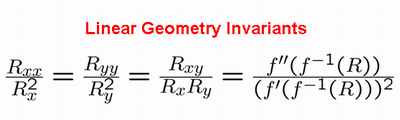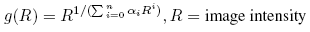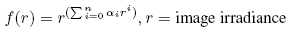Using Geometry Invariants for Camera Response Function EstimationWe propose a method for estimating the camera response function (CRF) from a single-channel image by using geometry invariants (shown in the figure above). The idea is based on the observation that image gradient contains information about the camera response function. Geometry invariants are independent on the geometry of the locally planar regions in image irradiance. These planar regions in image irradiance is detected using the constraint equation (shown in the figure above). We also propose a generalized gamma curve model (GGCM) which fits well on the real-world CRF's.

Publications [bibtex]

```"Using Geometry Invariants for Camera Response Function Estimation"
Tian-Tsong Ng, Shih-Fu Chang, Mao-Pei Tsui
IEEE Conference on Computer Vision and Pattern Recognition
2007
[Abstract][pdf]```

IllustrationsImage Gradient and Camera Response Function A typical camera response function has the property of compressing signal at the high irradiance region and expanding signal at the low irradiance region. This property can be captured by image gradient.Geometry Invariants From the observation that image gradient contains information about the camera response function, we obtain geometry invariants. For the planar regions in image irradiance, geometry invariant is only a function of the camera response function but not the geometry of the image irradiance.Geometric Interpretation of the Geometry Invariant Constraint Equation The intensity function that satisfies the goemetry invariant constraint equation is one with locally linear isophote (i.e., equal intensity profile).Steps in the CRF Estimation Algorithm There are three main steps in the CRF estimation Algorithm, as shown in the figure on the left.Selection of Locally Planar Irradiance Points (LPIP) Not all the points selected by the geometry invariant constraint equation are LPIP. We select LPIP's through Bayesian inference with feature independent assumption. The computed data performs well on simulation images after LPIP selection, as shown in the figure on the left.Generalized Gamma Curve Model (GGCM) We propose a curve model which offers a good fitting power to the real-world CRF's in the DoRF dataset.Estimation Camera Response Function (CRF) We estimate CRF through weighted least square error minimization. The minimization formulation is flexible in the sense that we can estimate CRF using one or more single channel images which share the same CRF. The plots on the left show the estimation results respectively using one, two and four single-channel image(s). Estimation is more accurate when more images are available.